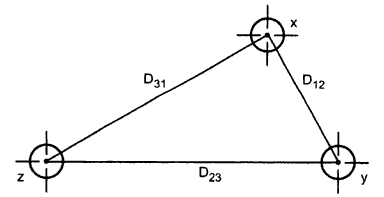### Inductance of Three Phase Lines with Unsymmetrical Spacing but Transposed

Consider a three phase line having three conductors but not spaced equilaterally. The problem of finding the inductance in this case is difficult. The flux linkage and the corresponding inductance will not be same in each phase. Due to this different inductance per phase there is unbalance in the circuit through the currents in each phases are balanced.
The drops in the three phases due to these inductance are observed to be different. Thus at the receiving end we will not get the same voltage.
In order to achieve balance under this case, transposition of transmission line is preferred after a certain fixed distance. This is shown in the Fig. 1.Fig. 1
The positions of the conductors are exchanged at regular interval along the line so that each conductor occupies the original position of every other conductor over an equal distance. This exchange of conductor positions is called transposition. Thus balance in the three phase is restored.
The Fig. 2 shows complete transposition cycle. The conductors in the individual phases are denoted by x, y and z where the positions are given by 1, 2, and 3. The same average inductance over the complete cycle is obtained due to the transposition.Fig. 2
The average inductance of one conductor is obtained by finding the flux linkages of a conductor for each position that is occupied during a complete cycle of transposition. Then the average flux linkages are obtained.
Now let us find the flux linkages of conductor x which is in position 1 whereas conductor y and z are in positions 2 and 3 respectively.
Conductor x is in position 2 whereas conductors y and z are in positions 3 and 1 respectively.
Conductor x is in position 3 whereas conductor y and z are in position 1 and 2 respectively.
The average value of flux linkage of conductor x is
But we have  Ix +  Iy +  Iz = 0
...                         Iy + Iz = - Ix
Thus the average inductance per phase is
In modern power lines, transposition of lines is not done at regular intervals even though an exchange in conductor positions can be made at switching stations to balance the inductance per phase. The inequality in the phases of an untransposed line is small and neglected in many cases.
If the dissymmetry is neglected, the inductance of the untransposed line is the average value of the inductive reactance of one phase of the same line correctly transposed.

1.